# Day Number of the Year – Formula for Excel or Google Sheets

This tutorial will demonstrate how to calculate a date’s day number of the year or find the nth day of the year in Excel and Google Sheets.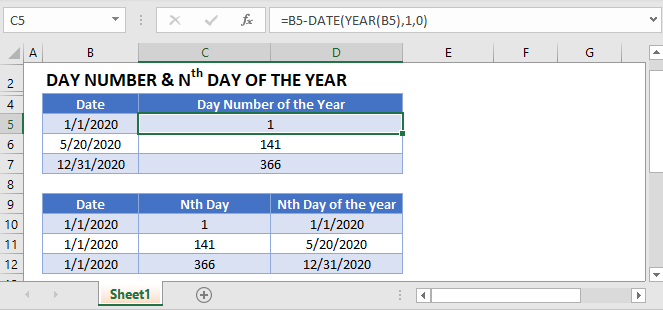## Date’s Day Number of the Year

To calculate a date’s day number of the year you can calculate the difference between the date and the first day of the year:

``=B3-DATE(YEAR(B3),1,1)+1``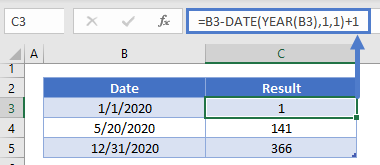Notice we need to add 1 to the end of the formula to calculate the correct day number. Instead we can set the day to 0 in the Date Function:

``=B3-DATE(YEAR(B3),1,0)``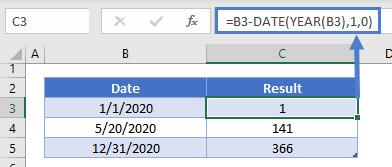## nth Day of the Year

Conversely you can calculate the nth day of the year by adding n days to 12/31 of the previous year:

``=DATE(2020,1,0)+C3``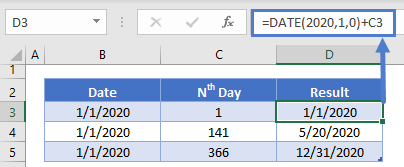Notice we do this by once again setting day =0 in the Date Function.

## Today’s Day Number

Use the TODAY Function to calculate today’s day number:

``= TODAY() - DATE(YEAR(TODAY()),1,0)``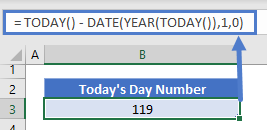## Google Sheets – Day Number Of The Year

All of the above examples work exactly the same in Google Sheets as in Excel.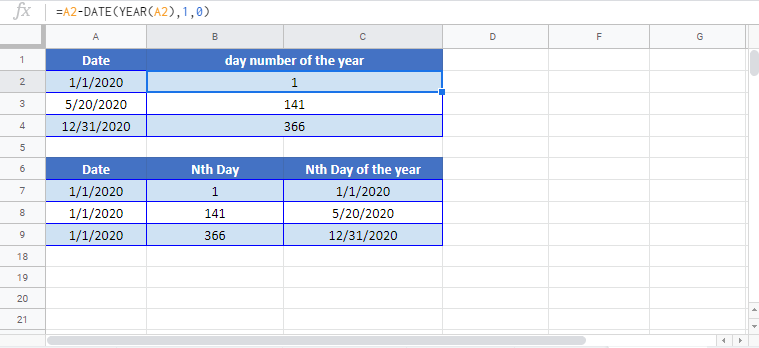### Excel Practice Worksheet

Practice Excel functions and formulas with our 100% free practice worksheets!